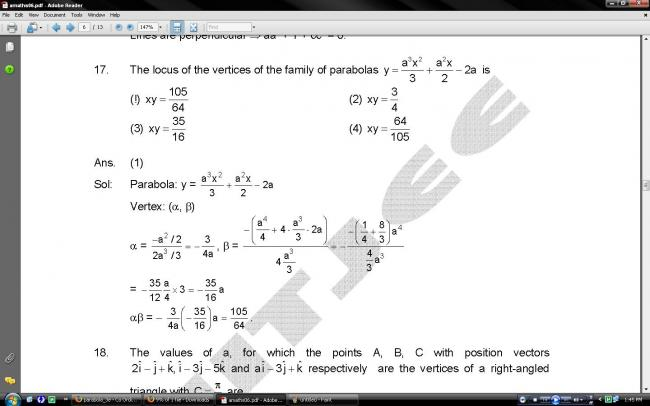# parabola ->3e

Q
17. The locus of the vertices of the family of parabolas y=a3x2/3+ a2x- 2a is
(1) xy= 105/64
(2) xy= 3/4
(3) xy= 35/16
(4) xy=64/105

•Mani Pal Singh ·

sir answer to yeh diya hua hai
but didn't understood it•Dharun ·

mani i am getting XY=(33/8)

•Son Goku ·

oh yeah mani pal singh is ryt

•Mani Pal Singh ·

bhai ai3e ka ques hai 

•Mani Pal Singh ·

N Y 1

•Lokesh Verma ·

17. The locus of the vertices of the family of parabolas y=a3x2/3+ a2x- 2a is
(1) xy= 105/64
(2) xy= 3/4
(3) xy= 35/16
(4) xy=64/105

y=a3x2/3+ a2x- 2a

The vertex will be the point where dy/dx = 0 (Think of it as a parabola)

2a3x/3+ a2 = 0

so x=-3/2a

there, y= ax3/4 - ax3/2 -2a = -11/4 a

thus, xy=33/8 should be the answer

•Dharun ·

they use partial differenciation method

bt its wrong fr β

•Dharun ·

sorry i don't knew hindi

•Mani Pal Singh ·

dude i am not saying that sir and u r wrong
but ur answer is not even in the options

then wat to do?

•Dharun ·

i am saying that soln u gvn is wrong

there r some ques like this its very few

•